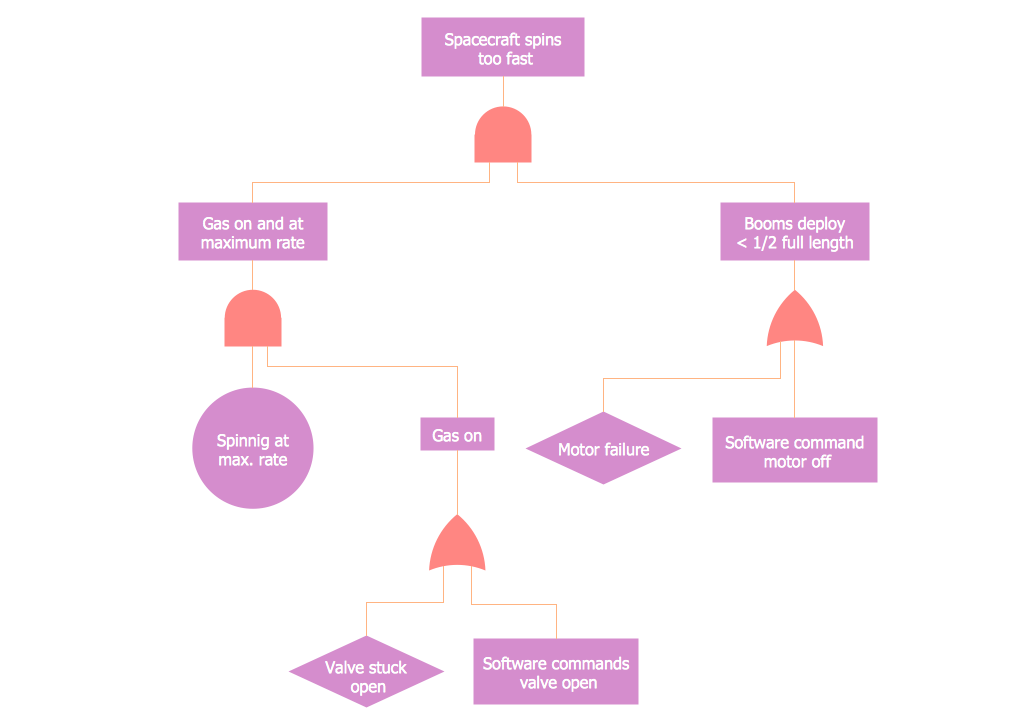This site uses cookies. By continuing to browse the ConceptDraw site you are agreeing to our Use of Site Cookies.
Engineering — Fault tree analysis diagrams

# Fault Tree Analysis Diagrams

Samples of fault tree analysis (FTA) diagrams are created using ConceptDraw DIAGRAM diagramming and vector drawing software enhanced with Fault Tree Analysis Diagrams solution from ConceptDraw Solution Park.

## Tutorials and Solutions:

Video Tutorials: ConceptDraw Solution Park

Solutions: Fault Tree Analysis Diagrams for ConceptDraw DIAGRAM

## Sample 1: Fault Tree Analysis Diagram

Fault Tree Analysis Diagrams sample: Fault tree analysis diagram.

This example is created using ConceptDraw DIAGRAM diagramming software enhanced with Fault Tree Analysis Diagrams solution from ConceptDraw Solution Park.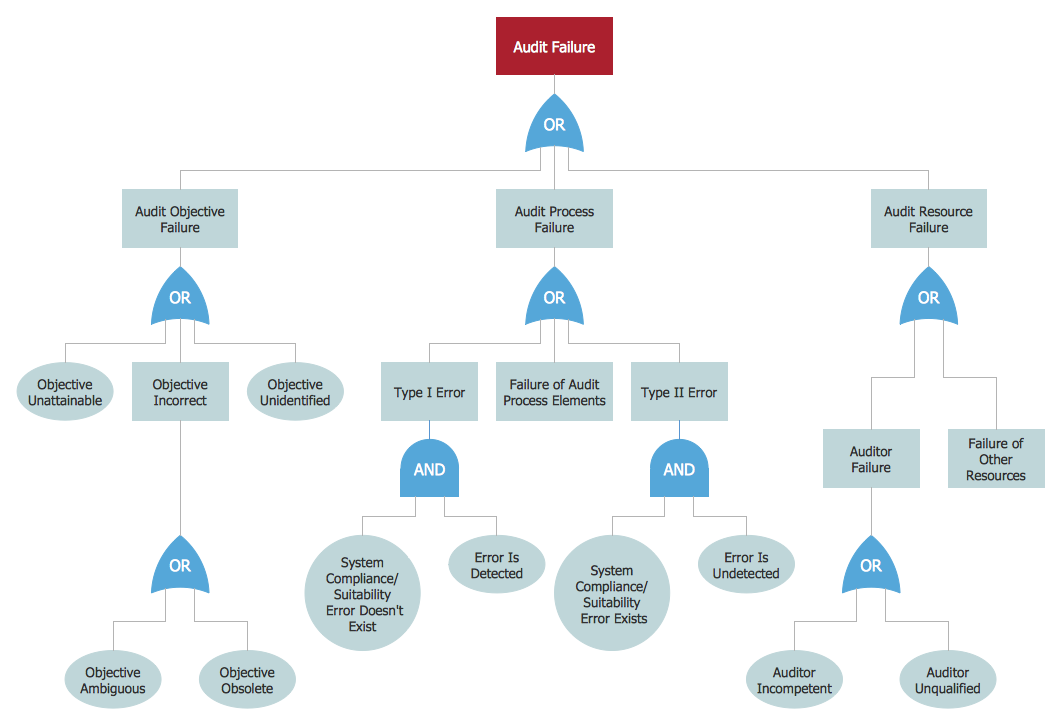## Sample 2: Fault Tree Diagram

Fault Tree Analysis Diagrams sample: Fault tree diagram.

This example is created using ConceptDraw DIAGRAM diagramming software enhanced with Fault Tree Analysis Diagrams solution from ConceptDraw Solution Park.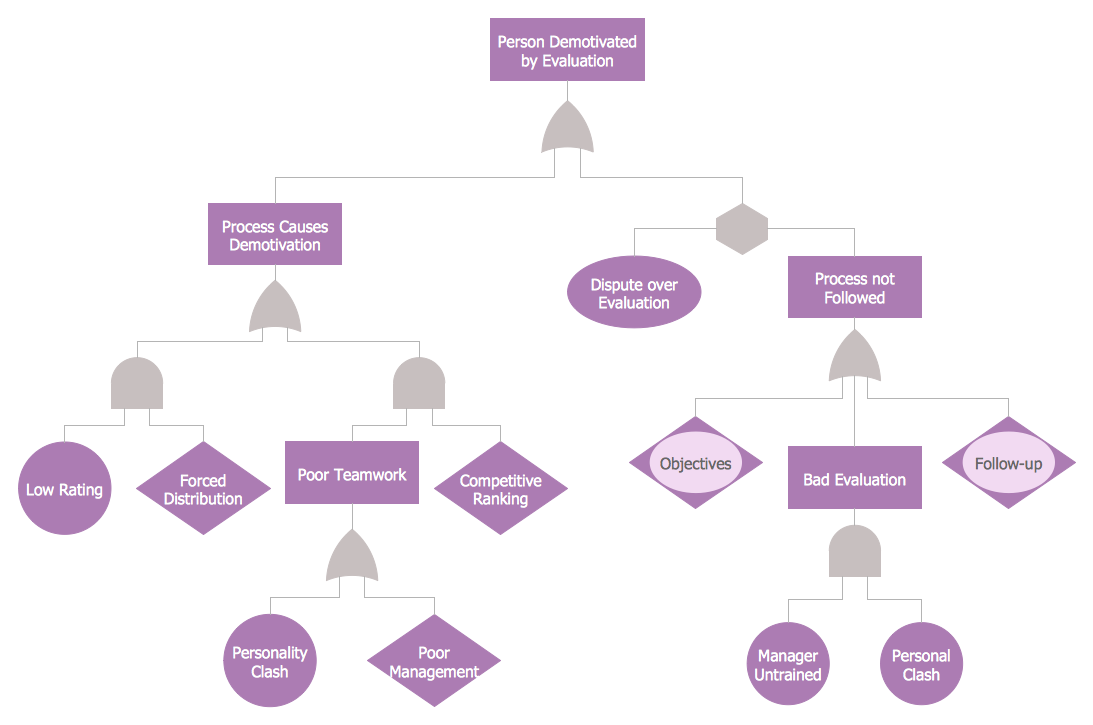## Sample 3: FTA Diagram — Accident Analytic Tree

Fault Tree Analysis Diagrams sample: Accident analytic tree.

This example is created using ConceptDraw DIAGRAM diagramming software enhanced with Fault Tree Analysis Diagrams solution from ConceptDraw Solution Park.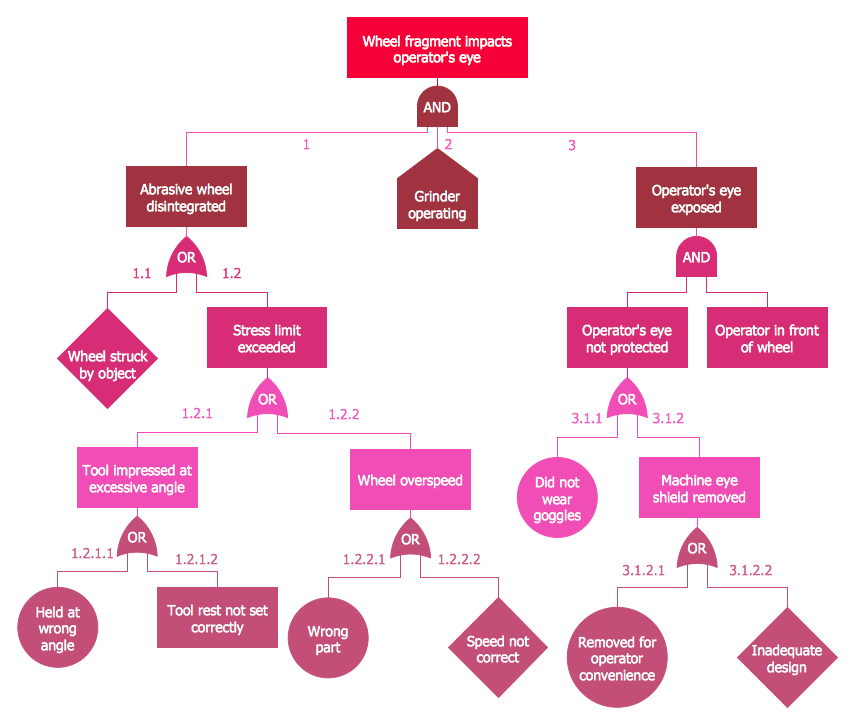## Sample 4: Fault Tree Analysis Diagram — Code Fault Tree

Fault Tree Analysis Diagrams sample: Code fault tree.

This example is created using ConceptDraw DIAGRAM diagramming software enhanced with Fault Tree Analysis Diagrams solution from ConceptDraw Solution Park.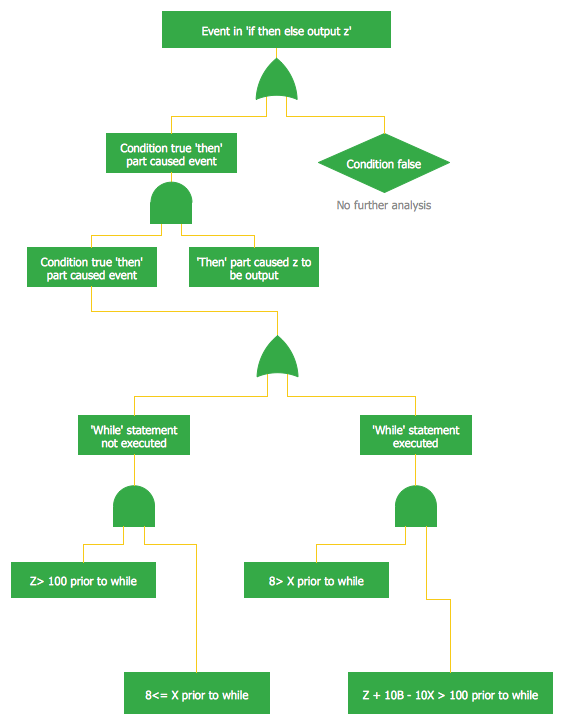## Sample 5: Fault Tree Analysis — Insulin Delivery System

Fault Tree Analysis Diagrams sample: Insulin delivery system.

This example is created using ConceptDraw DIAGRAM diagramming software enhanced with Fault Tree Analysis Diagrams solution from ConceptDraw Solution Park.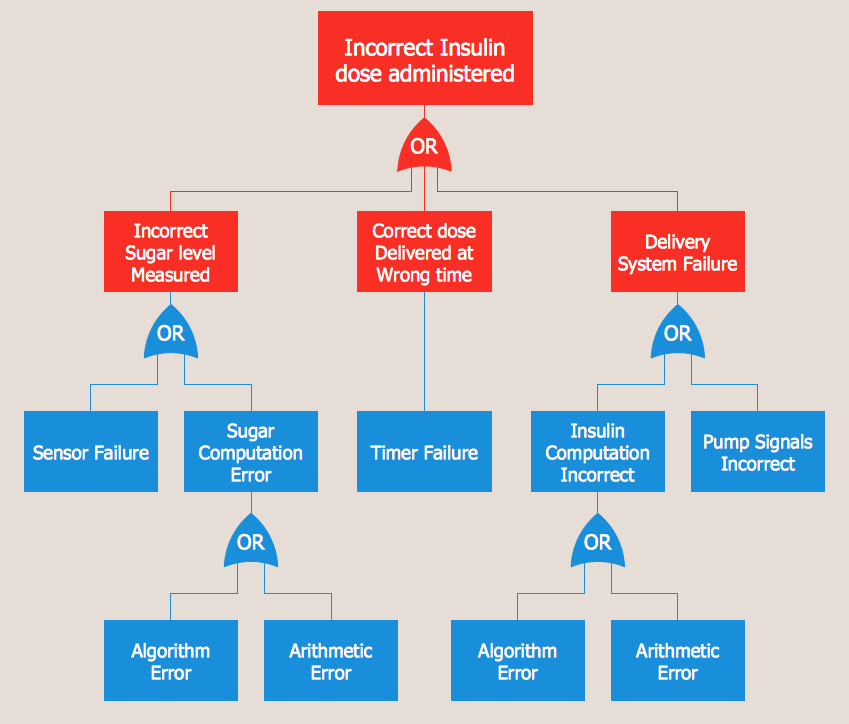## Sample 6: Fault Tree Analysis Diagram — Hazard Analysis

Fault Tree Analysis Diagrams sample: Hazard analysis.

This example is created using ConceptDraw DIAGRAM diagramming software enhanced with Fault Tree Analysis Diagrams solution from ConceptDraw Solution Park.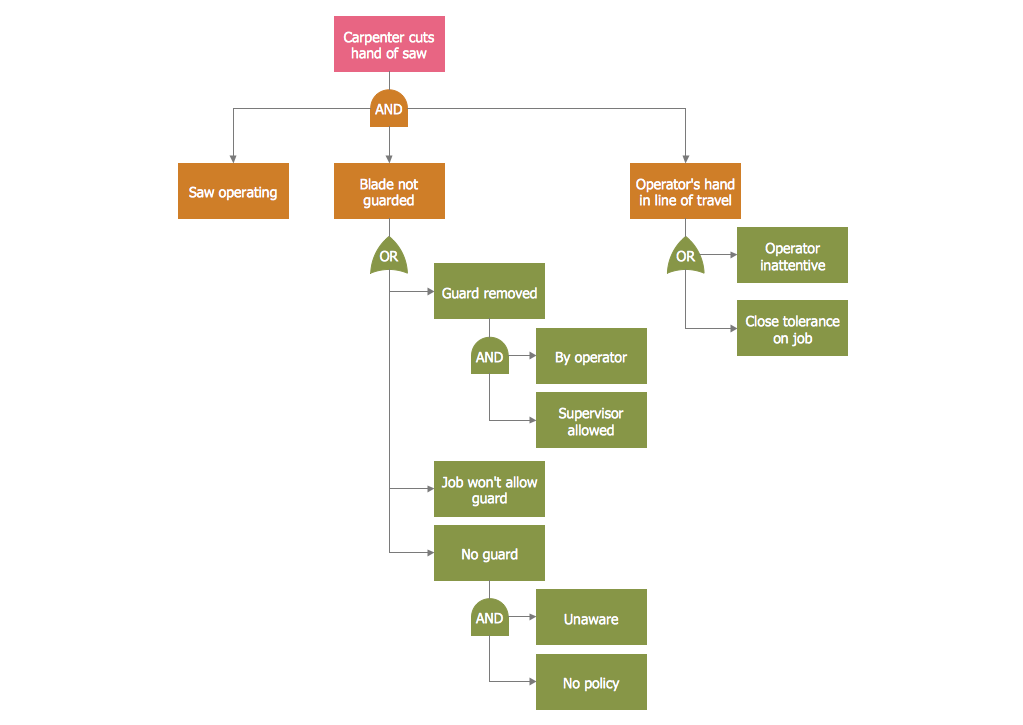## Sample 7: Fault Tree Analysis Diagram — High Level Fault Tree

Fault Tree Analysis Diagrams sample: High level fault tree.

This example is created using ConceptDraw DIAGRAM diagramming software enhanced with Fault Tree Analysis Diagrams solution from ConceptDraw Solution Park.